1596240720

# A Comprehensive Guide To Loss Functions  : Regression

Loss functions are used to calculate the difference between the predicted output and the actual output. To know how they fit into neural networks, read :

## Artificial Neural Networks: Explained

Typically when we say Neural Network, we are referring to Artificial Neural Networks (ANN). And though they may sound…

medium.com

## Let’s begin, shall we?

Loss functions are fundamentally dependent on the nature of our dependent variables and so, to select a loss function, we must examine if our dependent variables are numeric (in regression task) or probabilistic (in a classification task).

# Loss functions for regression :

When we are dealing with numeric variables, we have to measure the losses numerically, meaning, just knowing if the predicted value is wrong is not enough, we have to calculate the amount of deviation of our prediction from the actual value, so we can train our network accordingly.

The different loss functions for this are :

• Mean Absolute Error (MAE).
• Mean Absolute Percentage Error (MAPE).
• Mean Squared Error (MSE).
• Root Mean Squared Error (RMSE).
• Huber Loss.
• Log-Cosh Loss.

# Mean Absolute Error (MAE) :

MAE is the simplest error function, it literally just calculates the absolute difference (discards the sign) between the actual and predicted values and takes it’s mean.

## Mathematical Equation :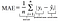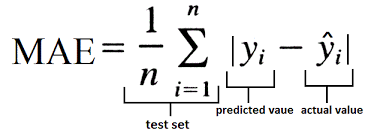MAE Equation from Data Vedas

## Graph :

The following figure shows that the MAE increases linearly with an increase in error.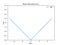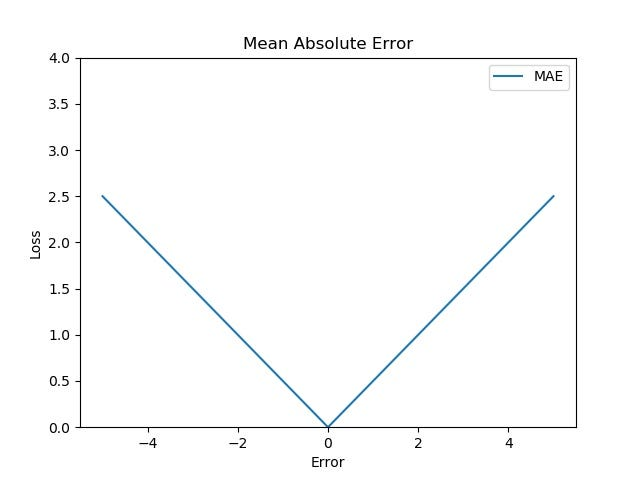Image by author

1. MAE is the simplest method to calculate the loss.
2. Due to its simplicity, it is computationally inexpensive.

## Drawbacks :

1. MAE calculates loss by considering all the errors on the same scale. For example, if one of the output is on the scale of hundred while other is on the scale of thousand, our network won’t be able to distinguish between them just based on MAE, and so, it’s hard to alter weights during backpropagation.
2. MAE is a linear scoring method, i.e. all the errors are weighted equally while calculating the mean. This means that while backpropagation, we may just jump past the minima due to MAE’s steep nature.

# Mean Absolute Percentage Error (MAPE) :

MAPE is similar to that of MAE, with one key difference, that it calculates error in terms of percentage, instead of raw values. Due to this, MAPE is independent of the scale of our variables.

## Mathematical Equation :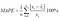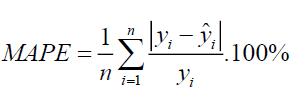MAPE Equation from JIBC

## Graph :

The following figure shows that the MAPE also increases linearly with an increase in error.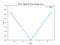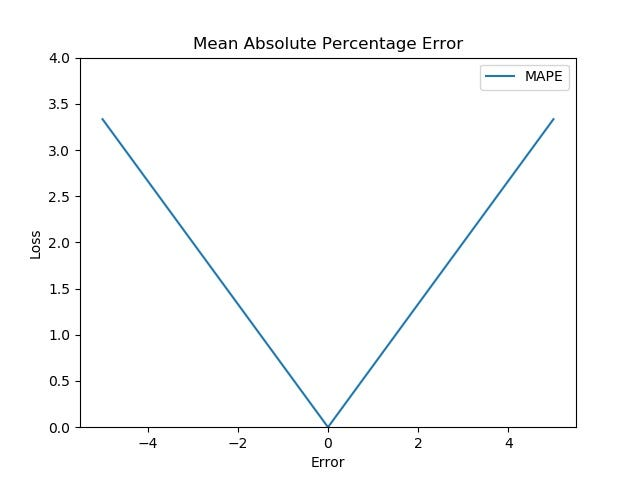Image by author

1. Loss is calculated by normalizing all errors on a common scale (of hundred).

1. MAPE equation has the expected output in the denominator, which can be zero. Loss cannot be calculated for these, as division by zero is not defined.
2. Again, division operation means that even for the same error, the magnitude of actual value can cause a difference in loss. For example, if the predicted value is 70 and the actual value is 100, the loss would be 0.3 (30%), while for the actual value of 40, the loss would be 0.75 (75%), even though the error in both the cases is the same, i.e. 30.

# Mean Squared Error (MSE) :

In MSE, we calculate the square of our error and then take it’s mean. This is a quadratic scoring method, meaning, the penalty is proportional to not the error (like in MAE), but to the square of the error, which gives relatively higher weight (penalty) to large errors/outliers, while smoothening the gradient for smaller errors.

#machine-learning #loss-function #deep-learning #artificial-intelligence #deep learning

## Buddha Community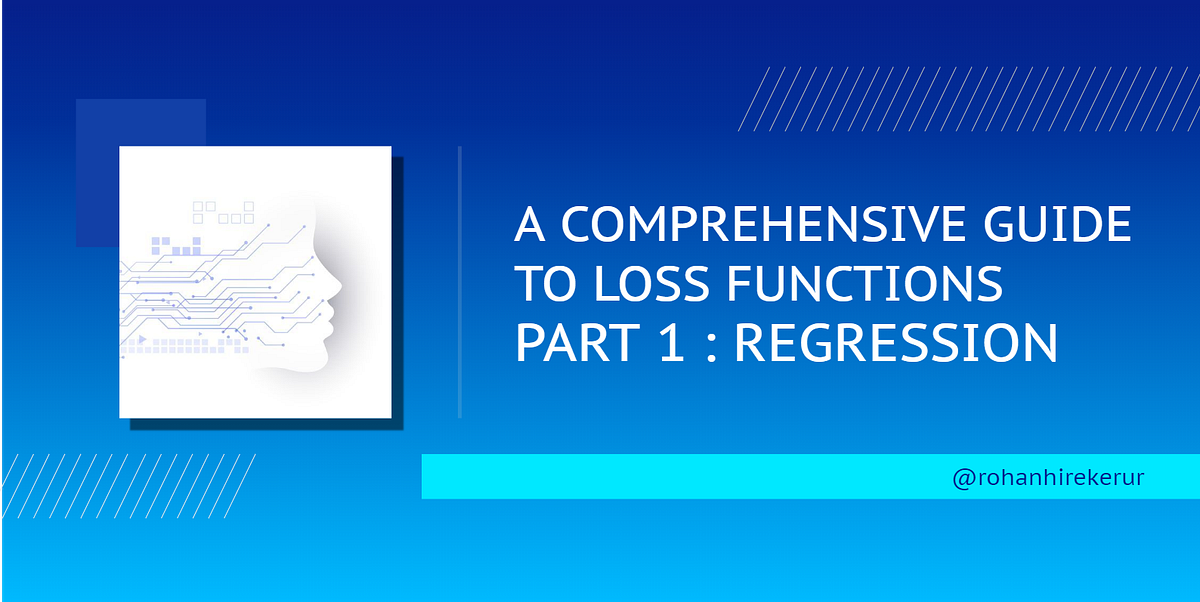1598258520

## Activation Functions:

A significant piece of a neural system Activation function is numerical conditions that decide the yield of a neural system. The capacity is joined to every neuron in the system and decides if it ought to be initiated (“fired”) or not, founded on whether every neuron’s info is applicable for the model’s expectation. Initiation works likewise help standardize the yield of every neuron to a range somewhere in the range of 1 and 0 or between — 1 and 1.

Progressively, neural systems use linear and non-linear activation functions, which can enable the system to learn complex information, figure and adapt practically any capacity speaking to an inquiry, and give precise forecasts.

### Linear Activation Functions:

**Step-Up: **Activation functions are dynamic units of neural systems. They figure the net yield of a neural node. In this, Heaviside step work is one of the most widely recognized initiation work in neural systems. The capacity produces paired yield. That is the motivation behind why it is additionally called paired advanced capacity.

The capacity produces 1 (or valid) when info passes edge limit though it produces 0 (or bogus) when information doesn’t pass edge. That is the reason, they are extremely valuable for paired order studies. Every rationale capacity can be actualized by neural systems. In this way, step work is usually utilized in crude neural systems without concealed layer or generally referred to name as single-layer perceptions.

#machine-learning #activation-functions #loss-function #optimization-algorithms #towards-data-science #function1605017502

## The Difference Between Regular Functions and Arrow Functions in JavaScript

Other then the syntactical differences. The main difference is the way the this keyword behaves? In an arrow function, the this keyword remains the same throughout the life-cycle of the function and is always bound to the value of this in the closest non-arrow parent function. Arrow functions can never be constructor functions so they can never be invoked with the new keyword. And they can never have duplicate named parameters like a regular function not using strict mode.

###### Here are a few code examples to show you some of the differences
```this.name = "Bob";
const person = {
name: “Jon”,
```<span style="color: #008000">// Regular function</span>
func1: <span style="color: #0000ff">function</span> () {
console.log(<span style="color: #0000ff">this</span>);
},

<span style="color: #008000">// Arrow function</span>
func2: () =&gt; {
console.log(<span style="color: #0000ff">this</span>);
}
```
}
person.func1(); // Call the Regular function
// Output: {name:“Jon”, func1:[Function: func1], func2:[Function: func2]}
person.func2(); // Call the Arrow function
// Output: {name:“Bob”}```

###### The new keyword with an arrow function
```const person = (name) => console.log("Your name is " + name);
const bob = new person("Bob");
// Uncaught TypeError: person is not a constructor```

###### If you want to see a visual presentation on the differences, then you can see the video below:

#arrow functions #javascript #regular functions #arrow functions vs normal functions #difference between functions and arrow functions1596240720

## A Comprehensive Guide To Loss Functions  : Regression

Loss functions are used to calculate the difference between the predicted output and the actual output. To know how they fit into neural networks, read :

## Artificial Neural Networks: Explained

Typically when we say Neural Network, we are referring to Artificial Neural Networks (ANN). And though they may sound…

medium.com

## Let’s begin, shall we?

Loss functions are fundamentally dependent on the nature of our dependent variables and so, to select a loss function, we must examine if our dependent variables are numeric (in regression task) or probabilistic (in a classification task).

# Loss functions for regression :

When we are dealing with numeric variables, we have to measure the losses numerically, meaning, just knowing if the predicted value is wrong is not enough, we have to calculate the amount of deviation of our prediction from the actual value, so we can train our network accordingly.

The different loss functions for this are :

• Mean Absolute Error (MAE).
• Mean Absolute Percentage Error (MAPE).
• Mean Squared Error (MSE).
• Root Mean Squared Error (RMSE).
• Huber Loss.
• Log-Cosh Loss.

# Mean Absolute Error (MAE) :

MAE is the simplest error function, it literally just calculates the absolute difference (discards the sign) between the actual and predicted values and takes it’s mean.

## Mathematical Equation :MAE Equation from Data Vedas

## Graph :

The following figure shows that the MAE increases linearly with an increase in error.Image by author

1. MAE is the simplest method to calculate the loss.
2. Due to its simplicity, it is computationally inexpensive.

## Drawbacks :

1. MAE calculates loss by considering all the errors on the same scale. For example, if one of the output is on the scale of hundred while other is on the scale of thousand, our network won’t be able to distinguish between them just based on MAE, and so, it’s hard to alter weights during backpropagation.
2. MAE is a linear scoring method, i.e. all the errors are weighted equally while calculating the mean. This means that while backpropagation, we may just jump past the minima due to MAE’s steep nature.

# Mean Absolute Percentage Error (MAPE) :

MAPE is similar to that of MAE, with one key difference, that it calculates error in terms of percentage, instead of raw values. Due to this, MAPE is independent of the scale of our variables.

## Mathematical Equation :MAPE Equation from JIBC

## Graph :

The following figure shows that the MAPE also increases linearly with an increase in error.Image by author

1. Loss is calculated by normalizing all errors on a common scale (of hundred).

1. MAPE equation has the expected output in the denominator, which can be zero. Loss cannot be calculated for these, as division by zero is not defined.
2. Again, division operation means that even for the same error, the magnitude of actual value can cause a difference in loss. For example, if the predicted value is 70 and the actual value is 100, the loss would be 0.3 (30%), while for the actual value of 40, the loss would be 0.75 (75%), even though the error in both the cases is the same, i.e. 30.

# Mean Squared Error (MSE) :

In MSE, we calculate the square of our error and then take it’s mean. This is a quadratic scoring method, meaning, the penalty is proportional to not the error (like in MAE), but to the square of the error, which gives relatively higher weight (penalty) to large errors/outliers, while smoothening the gradient for smaller errors.

#machine-learning #loss-function #deep-learning #artificial-intelligence #deep learning1596436980

## Higher-Order Functions Beginners Should Be Familiar With.

Higher-order functions are functions that operate on other functions, either by taking them as arguments or by returning them.

There are a lot more higher order functions than what will be covered in this article, but these are good ones to get you up and running as a beginner. These standard array methods are `forEach()` , `filter()` , `map()` and `sort()` .

1. **forEach( ): **This is used when you want to operate on or interact with any element inside of an array. Basically works like the_ for loop._

N.B- I’d be using examples to illustrate each method so you can get a clearer picture, and also just printing to the console to keep the examples as simple and basic as possible.

Example: Lets say in an array of a group or friends, and we want to loop through that array and print to the console each element of that array.

Using a for loop ;

``````const friends = ['Toyin', 'Olumide', 'Fola', 'Tola'];

for ( let i=0; i < friends.length ; i++) {
cosole.log (friends[i])
};
``````

The action same as above can be achieved using the`forEach()` method as seen below;

``````const friends =  ['Toyin', 'Olumide', 'Fola', 'Tola'];

friends.forEach(function(name) {
console.log(name)
};
``````

What the `forEach()` method simply does is to take in a function as an argument and loop through each item in the array without using iteration[i].

This is really awesome when the ES6 arrow functions are used, our code is reduced to a single line that is clean and maintainable. As seen below:

``````const friends =  ['Toyin', 'Olumide', 'Fola', 'Tola'];

friends.forEach(name => console.log (name));
``````

2. **_filter( ) : _**Just like the name implies, it is used to filter out elements of an array that do not meet the conditions set in the callback function passed as an argument. The callback function passed to the `filter()` method accepts 3 parameters: `element``index`, and `array` , but most times only the `element `parameter is used.

**Example : **In an array showing a group of friends and their ages, lets say we want to print to the console the friends that can drink assuming the age limit for drinking is 18. Using a for loop without high order functions;

``````const friends = [
{name : 'Toyin', age: 24},
{name : 'Olumide', age: 14},
{name : 'Fola', age: 12},
{name : 'David', age: 42}
];
for ( let i=0 ; i<friends.length ; i++) {
if (friends[i].age > 18) {
console.log(`\${friends[i].name} can drink`);
}
};
``````

Now using the `filter()` method :

``````const friends = [
{name : 'Toyin', age: 24},
{name : 'Olumide', age: 14},
{name : 'Fola', age: 12},
{name : 'David', age: 42}
];
friends.filter (function (friend) {
if (friend.age > 18){
return true;
}
});
``````

#functional-programming #beginners-guide #javascript #higher-order-function #es5-vs-es6 #function1598352300

## Regression: Linear Regression

Machine learning algorithms are not your regular algorithms that we may be used to because they are often described by a combination of some complex statistics and mathematics. Since it is very important to understand the background of any algorithm you want to implement, this could pose a challenge to people with a non-mathematical background as the maths can sap your motivation by slowing you down.In this article, we would be discussing linear and logistic regression and some regression techniques assuming we all have heard or even learnt about the Linear model in Mathematics class at high school. Hopefully, at the end of the article, the concept would be clearer.

**Regression Analysis **is a statistical process for estimating the relationships between the dependent variables (say Y) and one or more independent variables or predictors (X). It explains the changes in the dependent variables with respect to changes in select predictors. Some major uses for regression analysis are in determining the strength of predictors, forecasting an effect, and trend forecasting. It finds the significant relationship between variables and the impact of predictors on dependent variables. In regression, we fit a curve/line (regression/best fit line) to the data points, such that the differences between the distances of data points from the curve/line are minimized.

#regression #machine-learning #beginner #logistic-regression #linear-regression #deep learning